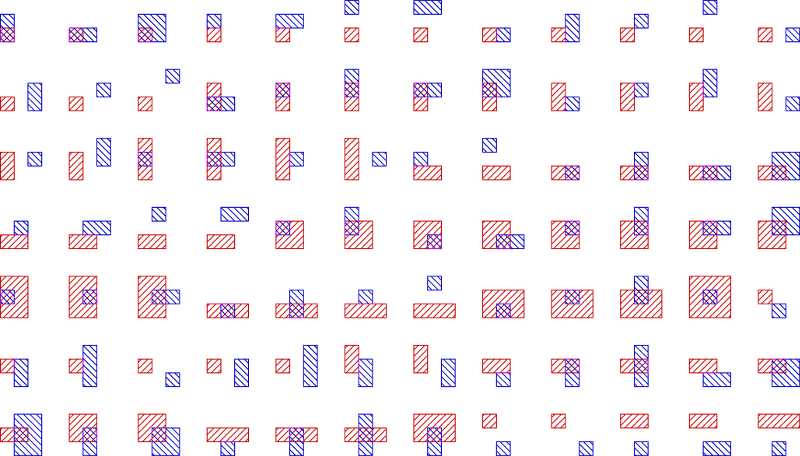# Internet Problem Solving Contest

## Problem C – Counting rectangles

• This problem has no input files.

This entire problem takes place in a standard two-dimensional plane.

Your task is very simple: count all fundamentally different sets of n axes-parallel rectangles.

Informally, two sets are fundamentally different if one cannot be transformed into the other by horizontally and vertically stretching some parts of the plane. A precise formal definition is given below.

For n = 2 there are 84 such sets. All of them are shown in the following figure. (The two rectangles are indistinguishable but in our figure we show one in red and the other in blue to make the figures where they overlap easy to read.)### Formal definitions

Given an ordered pair of points (P, Q), their relative position is rp(P, Q)=(sgn(P.x − Q.x),sgn(P.y − Q.y)). Hence, there are nine possible relative positions, including “Q is somewhere to the left and down of P”, “Q is exactly below P”, and “P and Q are the same point”.

An axes-parallel rectangle is a rectangle such that each of its sides has a positive length and lies parallel to one of the coordinate axes. For an axes-parallel rectangle R we will use R through R to denote its bottom left, bottom right, top left, and top right corner.

Two sets of axes-parallel rectangles $\cal S$ and $\cal T$ are called similar if there is a one-to-one map φ from $\cal S$ to $\cal T$ such that
$$\forall S_1, S_2\in{\cal S}:~ \forall i, j\in\{0,1,2,3\}:~ rp( S_1[i], S_2[j] ) = rp( \varphi(S_1)[i], \varphi(S_2)[j] )$$

In human words, if $\cal S$ and $\cal T$ each have n rectangles, we call them similar if we can number the rectangles in each set 1 through n in such a way that the relative positions of all their pairs of corners are the same in both cases.

### Problem specification

Given n, find the maximum number of sets of n rectangles such that no two sets are similar.

(Note that the order of rectangles in a set does not matter. Also note that sets cannot contain duplicates, hence the n rectangles in each set must be distinct.)

### Input specification

There is no input.

### Output specification

Let cn be the number of fundamentally different sets of n rectangles, and let $m_n = c_n \bmod (10^9 + 7)$.

For the easy subproblem C1 submit an output file with 4 lines, containing m1 through m4.

For the hard subproblem C2 submit an output file with 5000 lines, containing m1 through m5000.

### Example

Output for C1:

1
84
some_bigger_number
an_even_bigger_number